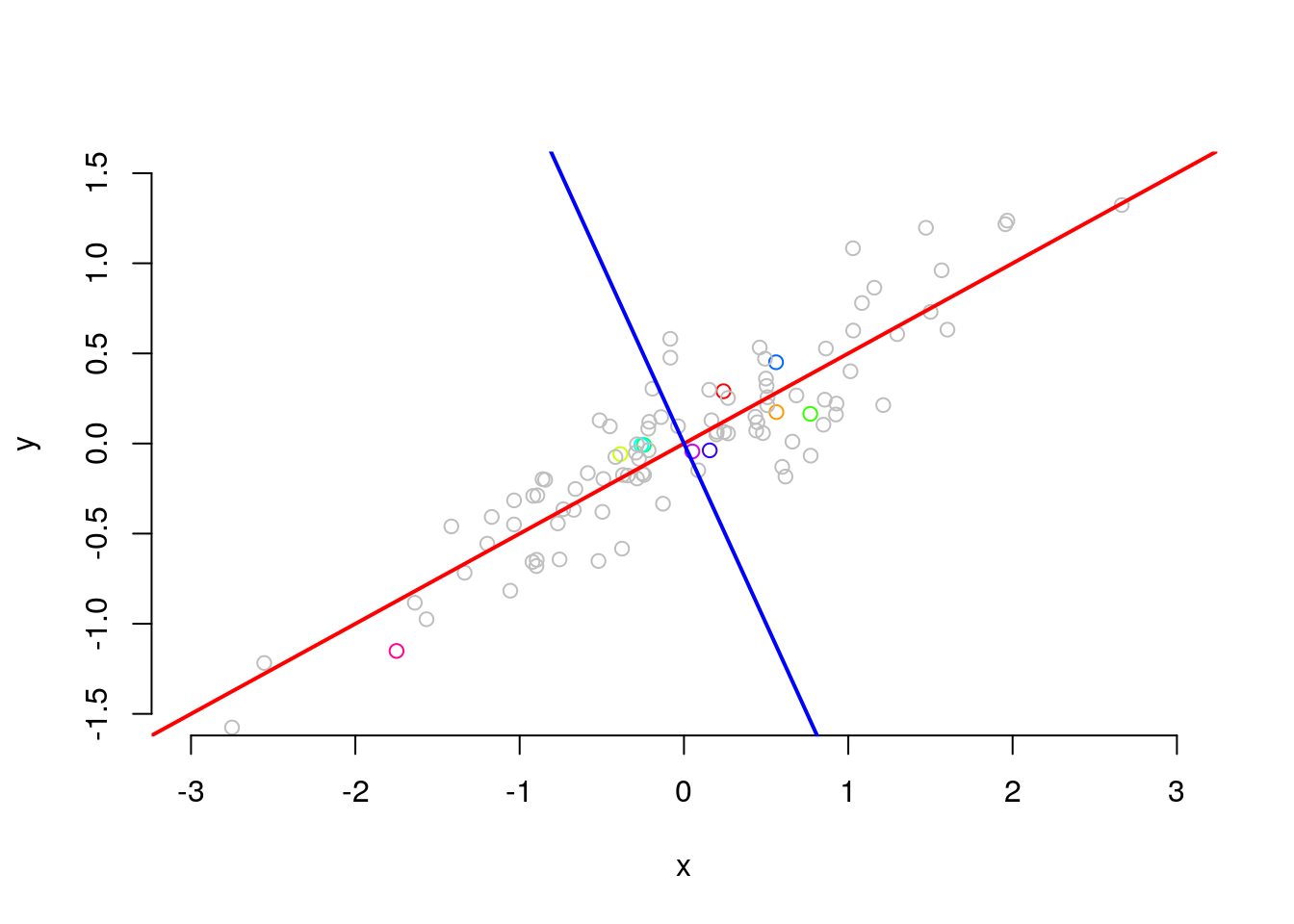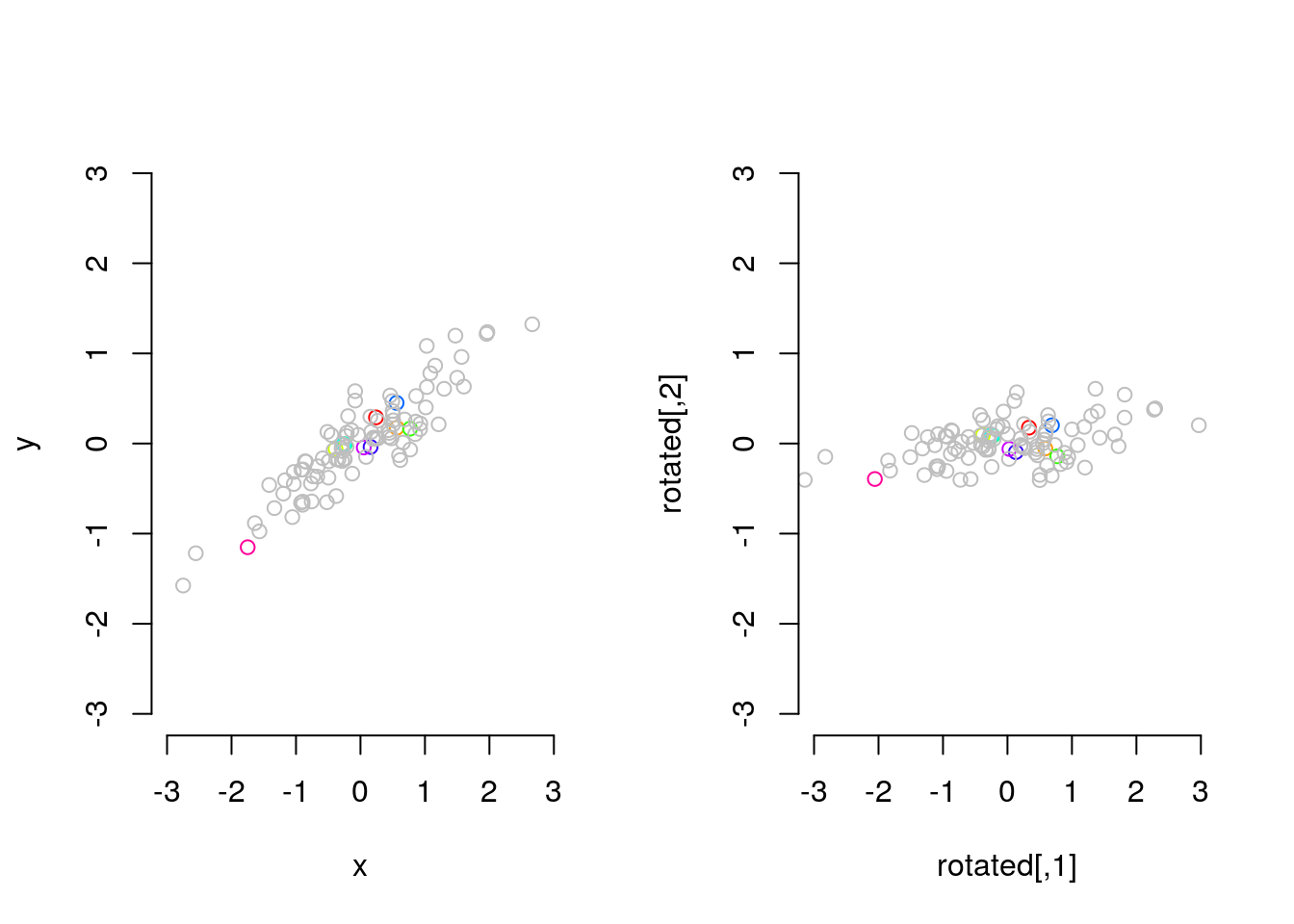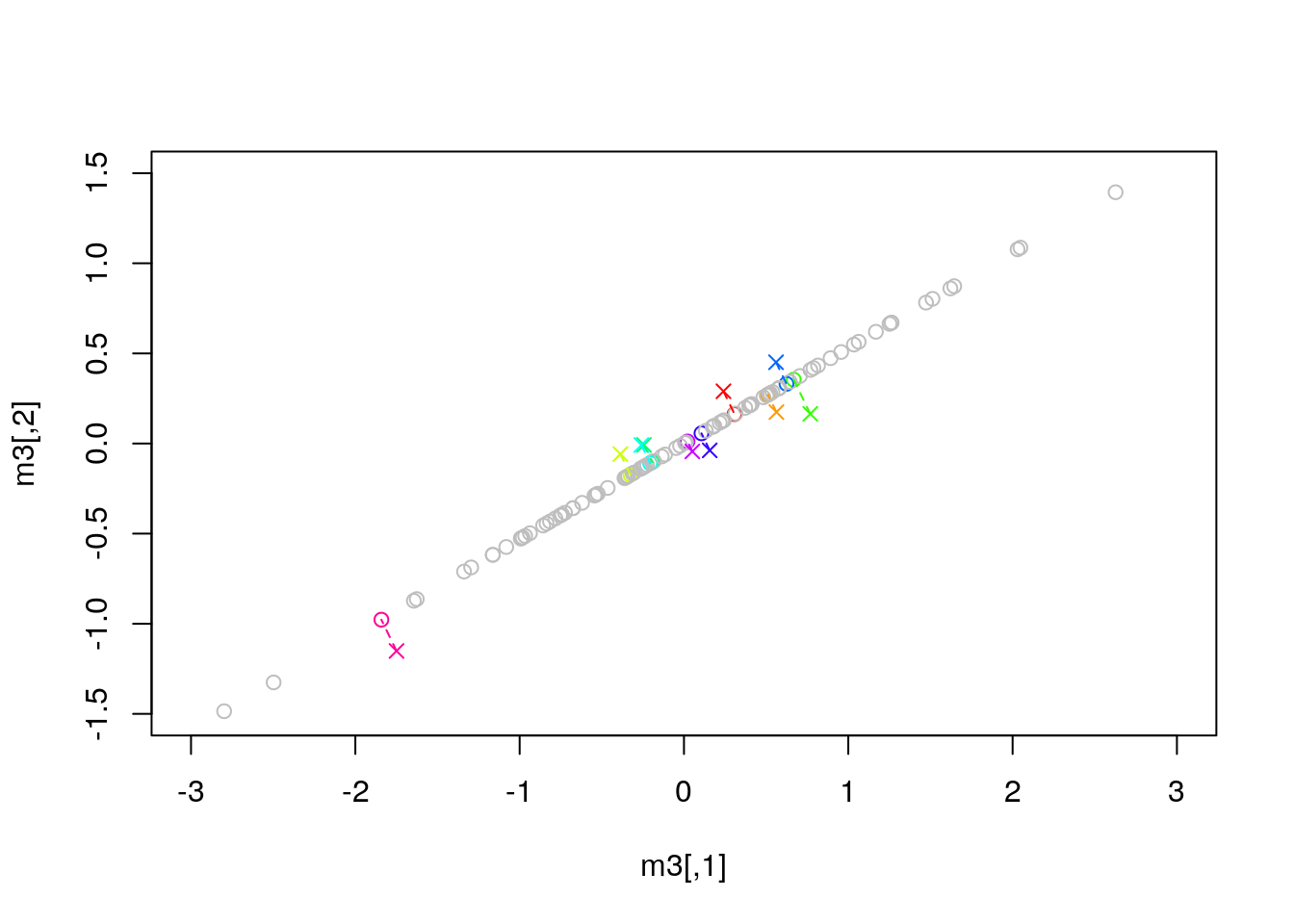# Graphical interpretation of Factor Analysis / SVD

This brief handout gives an idea of the way Factor Analysis and SVD reduce the dimensionality of a data set by transforming them into a space with only the most important (‘principal’) underlying components of the data.

This uses 2D data for ease of visualization, but the same idea holds in higher space: instead of fitting a line through points, you fit a (hyper)plane through a cloud of points.

# The Principal Components

Let’s generate some data with $$y = 0.5 x + \cal{N}(0, .25)$$

x = rnorm(100)
y = .5*x + rnorm(length(x), sd=.25)

Let’s plot this together with the (theoretical) regression line of 0.5 and a second line perpendicular to it:

colors = c(rainbow(10), rep("grey", 90))
plot(x,y, frame.plot = F, xlim=c(-3,3), ylim=c(-1.5,1.5), col=colors)
abline(coef = c(0, .5), col="red", lwd=2)
abline(coef = c(0, -2), col="blue", lwd=2)(I’m plotting a couple of points with colour to make it easier to see the same points in the transformed version)

As is obvious from the plot, most of the variation is along the regression intercept line of around .5. Any point can now also be expressed in terms of its coordinates along the red/blue axis instead of the x/y axis, in other words it can be projected on the new axis.

The advantage of the new dimensions is that much more of the variation is now along the first (blue) axis. In other words: the position on the blue axis contains more information about its position in the original space than the red axis. So, if you drop the red axis the main form of the data is the same, while if you would drop the y-axis in the original you would lose much more information.

You can see this projection as a rotation around the intercept by 22.5 degrees. You can rotate a dataset by multiplying it with a rotation matrix:

alpha = -.125*pi # 22.5 degrees clockwise
rotm <- matrix(c(cos(alpha),sin(alpha),-sin(alpha),cos(alpha)),ncol=2)
rotated = t(rotm %*% t(cbind(x,y)))
head(rotated)
##            [,1]        [,2]
## [1,]  0.3330065  0.17580249
## [2,]  0.5865394 -0.05425902
## [3,] -0.3798712  0.09427682
## [4,]  0.7731301 -0.14208057
## [5,] -0.2281786  0.08612474
## [6,] -0.2428606  0.09152933

The rotated matrix give the projections of the points on the new axes and are the (unnormalized) factor loadings of the original points. You can see that there is much less variance in this space, and it is more concentrated in the first dimension:

rotx = rotated[1,]
roty = rotated[2,]
c(var(x), var(y)) # old
##  0.8738551 0.2748364
c(var(rotx), var(roty)) # new
##  0.01235655 0.20531128

Finally, we can plot the rotated points to show that it is really a rotation of the original:

par(mfrow=c(1,2))
plot(x,y, frame.plot = F, xlim=c(-3,3), ylim=c(-3,3), col=colors)
plot(rotated, frame.plot = F, xlim=c(-3,3), ylim=c(-3,3), col=colors)# Singular Value Decomposition

In the example above we knew the principal components from the generative process. Normally, of course, we need to determine them from the data.

Singular Value Decomposition decomposes a matrix into $$U D V^T$$, where U give the factor loadings and D the singular values.

m = cbind(x,y)
udv = svd(m)
u = udv$u d = diag(udv$d)
vt = t(udv\$v)
head(u)
##             [,1]        [,2]
## [1,] -0.03323687  0.07190113
## [2,] -0.05523808 -0.05507809
## [3,]  0.03523801  0.06520137
## [4,] -0.07216995 -0.10783471
## [5,]  0.02089879  0.05389342
## [6,]  0.02224475  0.05729272

We can recreate the original matrix by (matrix) multiplying $$U$$, $$D$$, and $$V^T$$ again:

m2 = u %*% d %*% vt
all.equal(as.numeric(m), as.numeric(m2))
##  TRUE

Now if we drop the second component by setting the relevant singular value to zero and project back, we see what information is lost:

d[2,2] = 0
m3 = u %*% d %*% vt
plot(m3, col=colors, xlim=c(-3,3), ylim=c(-1.5,1.5))
# add the original 10 points and draw lines from original to transformed
points(m[1:10,], col=colors[1:10], pch=4)
segments(x0=m[1:10,1], y0=m[1:10,2], x1=m3[1:10,1], y1=m3[1:10,2], col=colors[1:10], lty = 2)The interpretation here is that the real ‘data’ is the position on this diagonal, treating the variance on the orthogonal axis as noise. This is similar to regression analysis, but (OLS) regression minimizes the vertical deviation, while SVD minimizes the orthogonal deviation. Also, regression normally ‘predicts’ one dimension based on the rest, while in SVD you normally have many more ‘dependent’ variables than ‘independent’ factors.